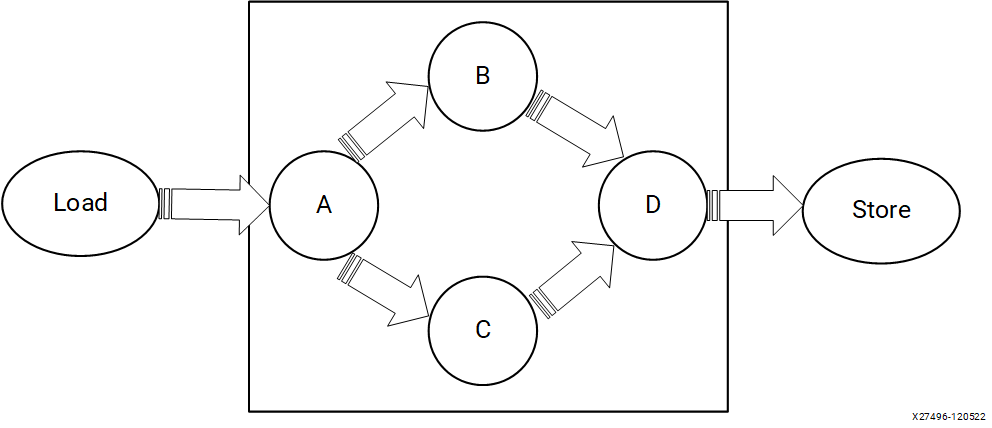# Re-Architecting the Design Code - 2022.2 English

## Vitis High-Level Synthesis User Guide (UG1399)

Document ID
UG1399
Release Date
2022-12-07
Version
2022.2 English

The following is a simple program that includes a `compute()` function written in C++ for execution on the CPU. The program is similar to any other C++ program where there the main function sets up the data to be sent to compute function, calls the compute function, and checks the results against expected results. The execution of this program is sequential on the CPU. This example will need to be re-architected to achieve significant performance improvement when running on programmable logic.

``````#include <vector>
#include <iostream>
#include <ap_int.h>
#include "hls_vector.h"

#define  totalNumWords 512
unsigned char data_t;

int main(int, char**) {
// initialize input vector arrays on CPU
for (int i = 0; i < totalNumWords; i++) {
in[i] = i;
}
compute(data_t in[totalNumWords], data_t Out[totalNumWords]);
check_results();
}

void compute (data_t in[totalNumWords ], data_t Out[totalNumWords ]) {
data_t tmp1[totalNumWords], tmp2[totalNumWords];
A: for (int i = 0; i < totalNumWords ; ++i) {
tmp1[i] = in[i] * 3;
tmp2[i] = in[i] * 3;
}
B: for (int i = 0; i < totalNumWords ; ++i) {
tmp1[i] = tmp1[i] + 25;
}
C: for (int i = 0; i < totalNumWords ; ++i) {
tmp2[i] = tmp2[i] * 2;
}
D: for (int i = 0; i <  totalNumWords ; ++i) {
out[i] = tmp1[i] + tmp2[i] * 2;
}
}``````

This program can also run sequentially on an FPGA, producing correct results without any performance gain compared to the CPU. For the application to execute with higher performance on an FPGA, the program needs to be re-architected to enable parallelism at various levels. Examples of parallelism can include:

• The compute function can start before all the data is transferred to it
• Multiple compute functions can run in an overlapping fashion, for example a "for" loop can start the next iteration before the previous iteration has completed
• The operations within a "for" loop can run concurrently on multiple words and doesn't need to be executed on a per-word basis

## Re-Architecting Kernel Code

From the prior example it is the `compute()` function that needs to be re-architected for FPGA-based acceleration.

The `compute()` function Loop A multiplies an input value with 3 and creates two separate paths, B and C. Loop B and C perform operations and feed the data to D. This is a simple representation of a realistic case where you have several tasks to be performed one after another and these tasks are connected to each other as a network like the one shown below.

Figure 1. Kernel ArchitectureThe key takeaways for re-architecting the kernel code are:

• Task-level parallelism is implemented at the function level. To implement task-level parallelism loops are pushed into separate functions. The original `compute()` function is split into multiple sub-functions. As a rule of thumb, sequential functions can be made to execute concurrently, and sequential loops can be pipelined.
• Instruction-level parallelism is implemented by reading 16 32-bit words from memory (or 512-bits of data). Computations can be performed on all these words in parallel. The `hls::vector` class is a C++ template class for executing vector operations on multiple samples concurrently.
• The `compute()` function needs to be re-architected into load-compute-store sub-functions, as shown in the example below. The load and store functions encapsulate the data accesses and isolate the computations performed by the various compute functions.
• Additionally, there are compiler directives starting with `#pragma` that can transform the sequential code into parallel execution.
Tip: This is the using_fifos example found in the Vitis-HLS Introductory Examples on GitHub.
``````#include "diamond.h"
#define NUM_WORDS 16
extern "C" {

void diamond(vecOf16Words* vecIn, vecOf16Words* vecOut, int size)
{
hls::stream<vecOf16Words> c0, c1, c2, c3, c4, c5;
assert(size % 16 == 0);

#pragma HLS dataflow
compute_A(c0, c1, c2, size);
compute_B(c1, c3, size);
compute_C(c2, c4, size);
compute_D(c3, c4,c5, size);
store(c5, vecOut, size);
}
}

void load(vecOf16Words *in, hls::stream<vecOf16Words >& out, int size)
{
Loop0:
for (int i = 0; i < size; i++)
{
#pragma HLS PERFORMANCE target_ti=32
#pragma HLS LOOP_TRIPCOUNT max=32
out.write(in[i]);
}
}

void compute_A(hls::stream<vecOf16Words >& in, hls::stream<vecOf16Words >& out1, hls::stream<vecOf16Words >& out2, int size)
{
Loop0:
for (int i = 0; i < size; i++)
{
#pragma HLS PERFORMANCE target_ti=32
#pragma HLS LOOP_TRIPCOUNT max=32
out1.write(t * 3);
out2.write(t * 3);
}
}
void compute_B(hls::stream<vecOf16Words >& in, hls::stream<vecOf16Words >& out, int size)
{
Loop0:
for (int i = 0; i < size; i++)
{
#pragma HLS PERFORMANCE target_ti=32
#pragma HLS LOOP_TRIPCOUNT max=32
}
}

void compute_C(hls::stream<vecOf16Words >& in, hls::stream<vecOf16Words >& out, int size)
{
Loop0:
for (data_t i = 0; i < size; i++)
{
#pragma HLS PERFORMANCE target_ti=32
#pragma HLS LOOP_TRIPCOUNT max=32
}
}
void compute_D(hls::stream<vecOf16Words >& in1, hls::stream<vecOf16Words >& in2, hls::stream<vecOf16Words >& out, int size)
{
Loop0:
for (data_t i = 0; i < size; i++)
{
#pragma HLS PERFORMANCE target_ti=32
#pragma HLS LOOP_TRIPCOUNT max=32